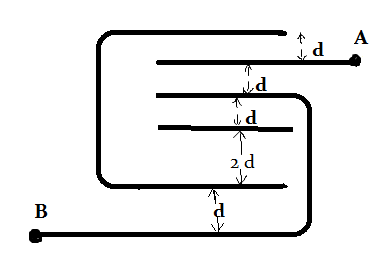# Tricky Capacitors

Find the equivalent capacitance between $A$ and $B$, $\dfrac{\epsilon A}{d}=7\text{ uF}$, where $A$ is area of plates with $A>>d$

Assume each conducting plate is having same dimensions and neglect the thickness of the plate.×

Problem Loading...

Note Loading...

Set Loading...